# Get minimum values in rows or columns with their index position in Pandas-Dataframe

## Get minimum values in rows or columns with their index position in Pandas-Dataframe

Let’s discuss how to find minimum values in rows & columns of a Dataframe and also their index position.

a) Find the minimum value among rows and columns :

Dataframe.min() : This function returns the minimum of the values in the given object. If the input is a series, the method will return a scalar which will be the minimum of the values in the series. If the input is a dataframe, then the method will return a series with a minimum of values over the specified axis in the dataframe. By default, the axis is the index axis.

1) Get minimum values of every column :
Use min() function to find the minimum value over the index axis.

Code :

 # import pandas library import pandas as pd   # list of Tuples data = [           (20, 16, 23),           (30, None, 11),           (40, 34, 11),           (50, 35, None),           (60, 40, 13)         ]   # creating a DataFrame object df = pd.DataFrame(data, index = ['a', 'b', 'c', 'd', 'e'],                      columns = ['x', 'y', 'z'])   # getting a series object containing  # minimum value from each column # of given dataframe minvalue_series = df.min()   minvalue_series

Output: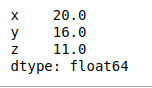2) Get minimum values of every row :
Use min() function on a dataframe with ‘axis = 1’ attribute to find the minimum value over the row axis.

Code :

 # import pandas library import pandas as pd   # list of Tuples data = [           (20, 16, 23),           (30, None, 11),           (40, 34, 11),           (50, 35, None),           (60, 40, 13)         ]   # creating a DataFrame object df = pd.DataFrame(data, index = ['a', 'b', 'c', 'd', 'e'],                      columns = ['x', 'y', 'z'])   # getting a series object containing  # minimum value from each row # of given dataframe minvalue_series = df.min(axis = 1)   minvalue_series

Output: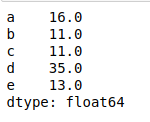3) Get minimum values of every column without skipping None Value :
Use min() function on a dataframe which has Na value with ‘skipna = False’ attribute to find the minimum value over the column axis.

Code :

 # import pandas library import pandas as pd   # list of Tuples data = [           (20, 16, 23),           (30, None, 11),           (40, 34, 11),           (50, 35, None),           (60, 40, 13)         ]   # creating a DataFrame object df = pd.DataFrame(data, index = ['a', 'b', 'c', 'd', 'e'],                      columns = ['x', 'y', 'z'])   # getting a series object containing  # minimum value from each column # of given dataframe without # skipping None value minvalue_series = df.min(skipna = False)   minvalue_series

Output: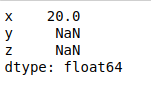4) Get minimum value of a single column :
Use min() function on a series to find the minimum value in the series.

Code :

 # import pandas library import pandas as pd   # list of Tuples data = [           (20, 16, 23),           (30, None, 11),           (40, 34, 11),           (50, 35, None),           (60, 40, 13)         ]   # creating a DataFrame object df = pd.DataFrame(data, index = ['a', 'b', 'c', 'd', 'e'],                      columns = ['x', 'y', 'z'])   # getting a minimum value # from column 'x' minvalue = df['x'].min()   minvalue

Output:

20

b) Get row index label or position of minimum values among rows and columns :

Dataframe.idxmin() : This function returns index of first occurrence of minimum over requested axis. While finding the index of the minimum value across any index, all NA/null values are excluded.

1) Get row index label of minimum value in every column :
Use idxmin() function to find the index/label of the minimum value along the index axis.

Code :

 # import pandas library import pandas as pd   # list of Tuples data = [           (20, 16, 23),           (30, None, 11),           (40, 34, 11),           (50, 35, None),           (60, 40, 13)         ]   # creating a DataFrame object df = pd.DataFrame(data, index = ['a', 'b', 'c', 'd', 'e'],                      columns = ['x', 'y', 'z'])   # get the index position\label of # minimum values in every column minvalueIndexLabel = df.idxmin()   minvalueIndexLabel

Output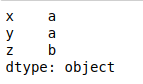2) Get Column names of minimum value in every row :
Use idxmin() function with ‘axis = 1’ attribute to find the index/label of the minimum value along the column axis.

Code :

 # import pandas library import pandas as pd   # list of Tuples data = [           (20, 16, 23),           (30, None, 11),           (40, 34, 11),           (50, 35, None),           (60, 40, 13)         ]   # creating a DataFrame object df = pd.DataFrame(data, index = ['a', 'b', 'c', 'd', 'e'],                      columns = ['x', 'y', 'z'])   # get the index position\label of # minimum values in every row minvalueIndexLabel = df.idxmin(axis = 1)   minvalueIndexLabel

Output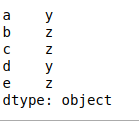Last Updated on March 1, 2022 by admin

## How to read multiple text files from folder in Python?How to read multiple text files from folder in Python?

How to read multiple text files from folder in Python? Python is a strong language

## Beautifulsoup Installation – PythonBeautifulsoup Installation – Python

Beautifulsoup Installation – Python Beautiful Soup is a Python library for pulling data out of

## Deploy Python Flask App on HerokuDeploy Python Flask App on Heroku

Deploy Python Flask App on Heroku Flask is a web application framework written in Python.

## Python OpenCV – cv2.polylines() methodPython OpenCV – cv2.polylines() method

Python OpenCV – cv2.polylines() method OpenCV is the huge open-source library for computer vision, machine

## Python String title() methodPython String title() method

Python String Title method The title() function in python is the Python String Method which

## Create XML Documents using PythonCreate XML Documents using Python

Create XML Documents using Python Extensible Markup Language(XML), is a markup language that you can

## Python IndentationPython Indentation

Indentation in Python Indentation is a very important concept of Python because without proper indenting